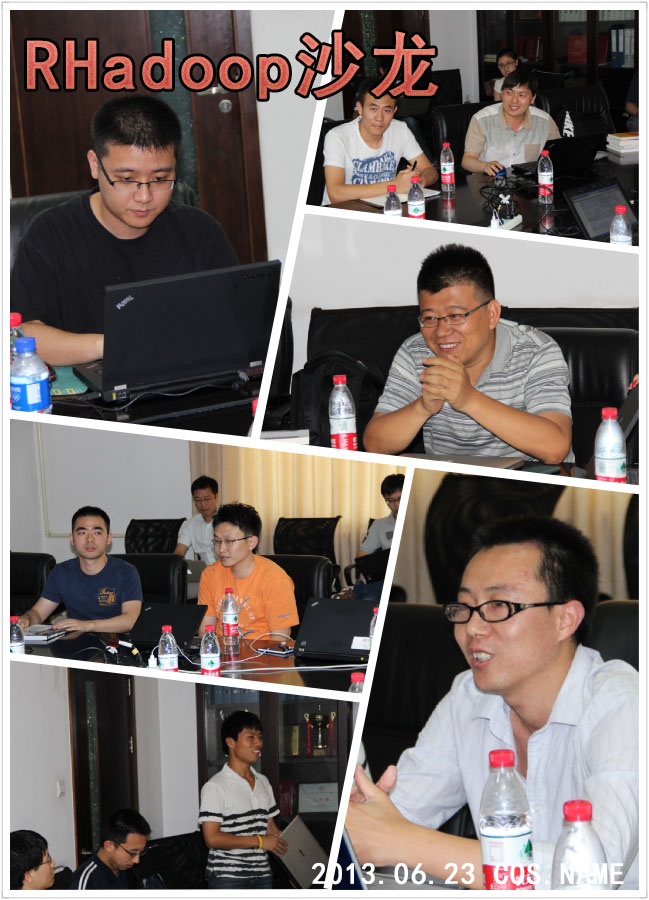#2013年6月23日，十一期COS数据分析沙龙（北京站）在明主1016如期举行。本期沙龙主题是“RHadoop助R突破大数据难关”；沙龙嘉宾张丹先生围绕沙龙主题分享了有关在ubuntu系统下配置RHadoop的相关知识以及基于RHadoop完成数据分析工作的相关案例。

RHadoop的命令与原生Hadoop命令相仿，只是为了调用方便做了一些封装。以rhdfs包为例。查看hdfs文件目录的Hadoop原生语句是：

hadoop fs -ls /user


hdfs.ls("/user/")


hadoop fs -cat /user/hdfs/o_same_school/part-m-00000


hdfs.cat("/user/hdfs/o_same_school/part-m-00000")


rmr2包是帮助R实现Map-Reduce算法的包，基于它我们可以做很多提高效率的事情。一个简单的例子是：

small.ints = 1:100000
sapply(small.ints, function(x) x^2)


small.ints= to.dfs(1:100000)
mapreduce(input = small.ints, map = function(k, v) cbind(v, v^2))
from.dfs("/tmp/RtmpWnzxl4/file5deb791fcbd5")


input <- '/user/hdfs/o_same_school/part-m-00000'
wordcount = function(input, output = NULL, pattern = " "){
wc.map = function(., lines) {
keyval(unlist( strsplit( x = lines, split = pattern)), 1)
}
wc.reduce = function(word, counts ) {
keyval(word, sum(counts))
}
mapreduce(input = input , output = output, input.format = "text",
map = wc.map, reduce = wc.reduce, combine = T)
}
wordcount(input)


RHadoop系列包的最后一个包是RHbase，它相当于是一个管理数据库的包。其包含的函数如下：

• hb.compact.table
• hb.describe.table
• hb.insert
• hb.regions.table
• hb.defaults
• hb.get
• hb.insert.data.frame
• hb.scan
• hb.delete
• hb.delete
• hb.get.data.frame
• hb.list.tables
• hb.scan.ex
• hb.delete.table
• hb.init
• hb.new.table
• hb.set.table.mode

• 建立物品的同现矩阵
• 建立用户对物品的评分矩阵
• 矩阵计算推荐结果

# RHbase

## 加载plyr包

library(plyr)


## 读取数据集

train <- read.csv(file = "small.csv", header = FALSE)
names(train) <- c("user", "item", "pref")


## 计算用户列表

usersUnique <- function(){
users <- unique(train$user) users[order(users)] }  ## 计算商品列表方法 itemsUnique <- function(){ items <- unique(train$item)
items[order(items)]
}


## 用户列表

users <- usersUnique()
users


## 商品列表

items <- itemsUnique()
items


## 建立商品列表索引

index <- function(x) which(items %in% x)
data <- ddply(train, .(user, item, pref), summarize, idx = index(item))


## 同现矩阵

cooccurrence <- function(data){
n <- length(items)
co <- matrix(rep(0, n * n),nrow = n)
for(u in users){
idx <- index(data$item[which(data$user == u)])
m <- merge(idx,idx)
for(i in 1:nrow(m)){
co[m$x[i], m$y[i]] = co[m$x[i], m$y[i]] + 1
}
}
return(co)
}


## 推荐算法

recommend <- function(udata = udata, co = coMatrix, num = 0){
n <- length(items) # all of pref
pref <- rep(0,n)
pref[udata$idx] <- udata$pref
## 用户评分矩阵
userx <- matrix(pref, nrow = n)
## 同现矩阵 * 评分矩阵
r <- co %*% userx
## 推荐结果排序
r[udata$idx] <- 0 idx <- order(r, decreasing = TRUE) topn <- data.frame(user = rep(udata$user, length(idx)),
item = items[idx], val = r[idx])
## 推荐结果取前num个
if(num > 0) topn <- head(topn, num)
## 返回结果
return(topn)
}


### 幻灯片下载

COS数据沙龙第9期幻灯片